# Introduction to Python - Lesson Four

Posted on 30-05-19 in Turtle Exercises

##### This post is part 4 of the Introduction to Programming" series
Introduction to Python - Lesson One

Introduction to programming using Python and Turtle

Introduction to Python - Lesson Two

Continuing our introduction to Python using Turtle

Introduction to Python - Lesson Three

Looking at loops and conditionals in more detail

Introduction to Python - Lesson Four

Further exploration of Turtle and an introduction to colour

Introduction to Python using Turtle : A Review

A brief review of the first part of this Turtle course

1. How to write functions
2. More Turtle methods including pen and fill colour
3. Feedback from Turtle

## Review

• Draw the following shapes
Hint! Try using a loop, inside a loop to alter the pen width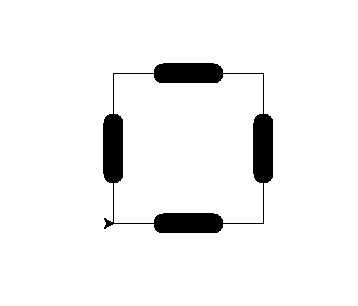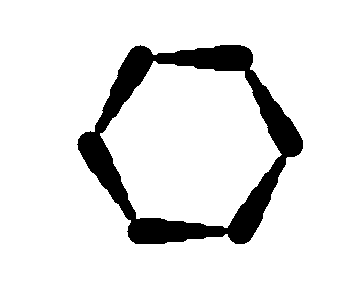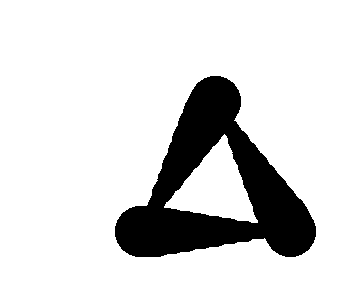Extension Segregate code for drawing each edge from drawing the regular polygon.

1. Without a computer, write out what numbers are printed in the following code :
```for j in range(5):
print(j)
```
```for x in range(5, 50, 5):
print(x)
```

## Using for loops with a list of numbers

In the last post we looked at using the range argument to produce sequences of numbers. For example `range(5,30,5)` will produce `5,10,15,20,25`. Note how it does not include the number `30`!

What if we want a list of numbers that isn't a pattern? We can do that!

```import turtle as tl

edge = 150

for angle in (45, 135, 45, 135):
tl.forward(edge)
tl.left(angle)

tl.exitonclick()
```

will produce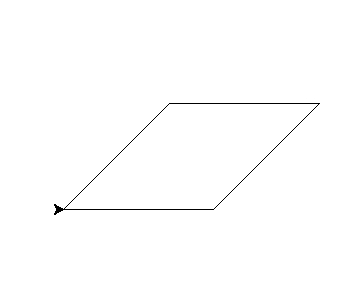We can also use this to produce patterns in the width like so

```import turtle as tl

edge = 150
sides = 6

for _ in range(sides):
for current_width in (2,4,8,4,2):
tl.width(current_width)
tl.forward(edge/5)
tl.left(360 / sides)

tl.exitonclick()
```

will produceIn both examples we have used a list of numbers, known as a tuple. This can be stored in a variable too.

```import turtle as tl

edge = 150
sides = 6
widths = (2,4,8,4,2)

for _ in range(sides):
for current_width in widths:
tl.width(current_width)
tl.forward(edge / len(widths))
tl.left(360 / sides)

tl.exitonclick()
```

Note how here we have used `len(widths)`. This returns the length, the number of items, in the list `widths`. Using such things we can design ever more complicated patterns!

Exercise Set 1

Draw the following patterns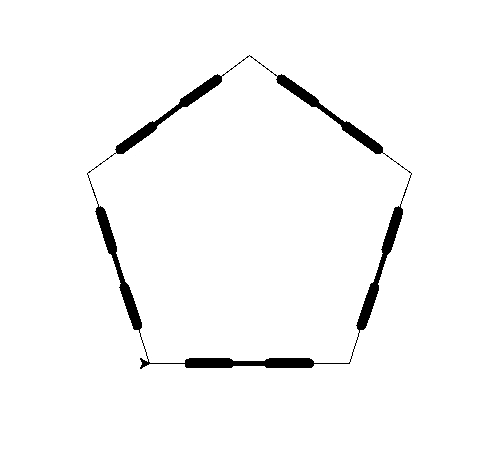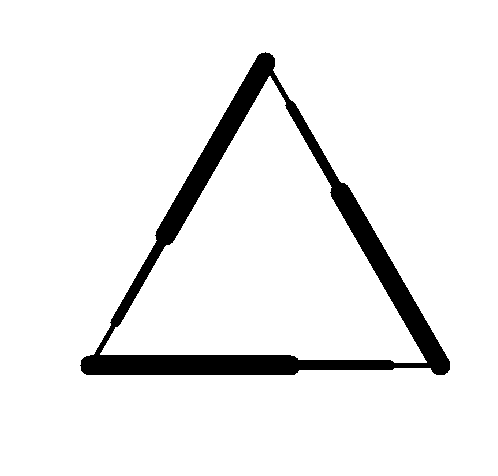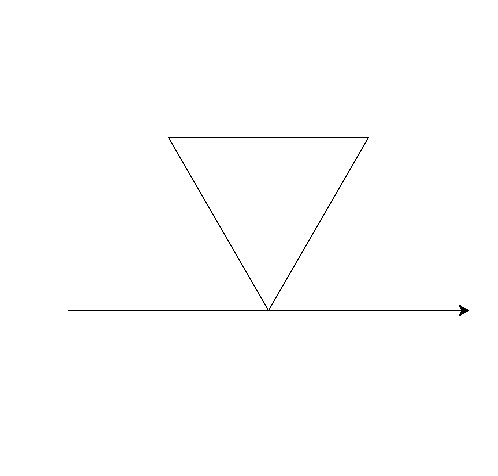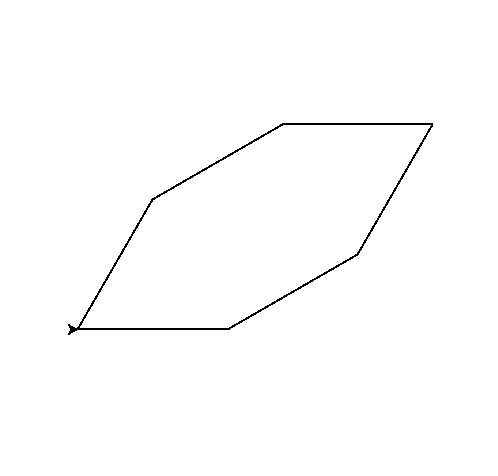## Introducing Colour

In Turtle we can use colours in two ways.

1. The pen colour - what colour the lines we draw will be
2. The fill colour - what colour closed shapes will be filled with

Let's consider pen colour first. Here is a triangle with three different colours.

```import turtle as tl

tl.colormode(255)
tl.width(10)

step = 200
angle = 120

colours = ((255,0,0), (0,255,0), (0,0,255))  # red, green then blue

for colour in colours:
tl.color(colour)
tl.forward(step)
tl.left(angle)

tl.exitonclick()
```

Here we have introduced two new methods - `colormode` and `color`. `colormode` tells turtle that each colour red, green and blue, gets a number from 0 to 255. `color` tells turtle what colour to set the pen. This is given as `(red, green, blue)`.

For example, `(255, 255, 255)` is all colours turned to maximum which is white. `(0,0,0)` is all colours set to minimum, which is black.

## Summary

In this class we have explored further properties of turtle including how computers use colour.